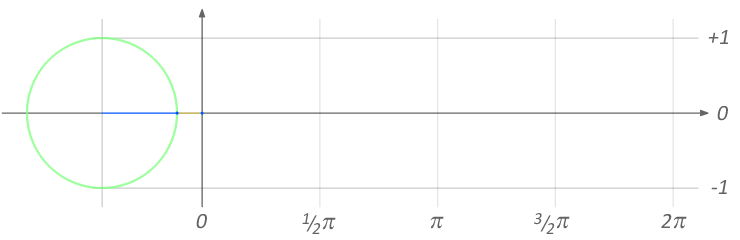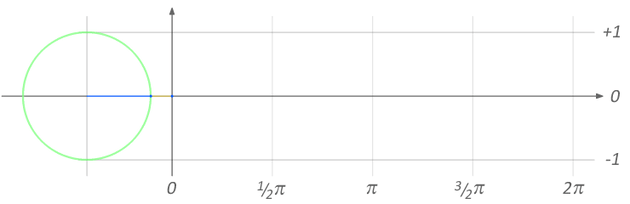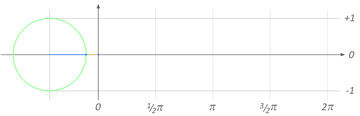Unlimited WordPress themes, graphics, videos & courses! Unlimited asset downloads! From \$16.50/m# Quick Tip: Create Smooth Enemy Movement With Sinusoidal Motion

In this Quick Tip, I'll show you how to use the sine function to give your game's objects smooth back-and-forth motion - no more harsh zig-zags where your floating enemies seem to bounce against an invisible wall!

## Examples

First, let me show you the kind of smooth back-and-forth motion I mean. (Graphics are from our totally free shoot-'em-up sprite pack.)

This enemy moves up and down, shooting bullets at regular intervals as it goes:

This enemy weaves across the screen:

Both types of movement are handy for shoot-'em-up games. Notice how smooth and gradual the motion feels - no sudden movements, no "jerk" as the enemy changes direction. That's in stark contrast to...

## The Naive Approach

A common first attempt at creating a back-and-forth motion is to do something like this:

Basically, this tells the enemy to move down at a constant rate (i.e. the same number of pixels each time) until it reaches the lowest point in its allowed range, then to move up at that same constant rate until it reaches the highest point in its allowed range, over and over again.

The enemy can be made to move horizontally by setting its xSpeed to any number other than zero: a negative number makes it move left, and a positive number makes it move right.

These examples show what this kind of motion looks like. First, with no horizontal motion:

Now, with horizontal motion:

It achieves the goal of moving back and forth, but it certainly isn't as smooth as our earlier example.

## The Cause

The reason for this bumpy motion is that the enemy's vertical speed makes a sudden huge change - even though the value of ufo.ySpeed stays the same.

Suppose ufo.ySpeed is 10. On the way up, the enemy is moving upwards at 10px/tick (pixels per tick, where a "tick" is the length of one game loop). Once the enemy reaches the top, it reverses direction, and is suddenly moving at 10px/tick downwards. The shift from +10px/tick to -10px/tick is a difference of 20px/tick, and that's what's so noticeable.

When the cause is spelled out like this, the solution seems obvious: slow the enemy down near the highest and lowest points! That way, the change in its speed won't be so big when it reverses direction.

A first attempt at this might look like this:

This code is messy, but you get the idea: if the enemy is within 100px of its highest or lowest boundaries, it moves at half of its normal speed.

This works, although it's not perfect. The enemy will still have a "jump" in speed when it changes direction, but at least it won't be as noticeable. However, the enemy will now have additional jumps in speed when it moves from its regular pace to the slower speed! Dang.

We could fix this by splitting the range into smaller sections, or making the speed some multiple of the exact distance from the enemy to its boundaries... but there's an easier way.

## Sinusoidal Motion

Think of a model train going around a perfectly circular track. The train is constantly changing direction, and yet it's moving at a steady pace, with no "jumps".

Now imagine a wall on one side of the circular track and a big bright light on the opposite side (so, the track and the train are in between the two). The train will cast a shadow on the wall. But of course that shadow won't move in a circle, because the wall is flat: it'll move back and forth, in a straight line, but still with that smooth jump-free motion of the train!

That's exactly what we want. And fortunately there's a function that will give it to us: the sine function. This animated GIF from Wikipedia demonstrates:Image from Wikimedia Commons. Thanks, Lucas!

The red line is the curve of y = sin(x). So, sin(0.5 * pi) is 1, sin(pi) is 0, and so on.

It's a little inconvenient that pi (π) is the basic unit used for this function, but we can manage. We can use it like so:

See what's happening here? After one tick, ufo.y will be set to sin(1 * pi), which is 0. After two ticks, ufo.y will be set to sin(2 * pi), which is... 0, again. Oh. Hang on.

Now, after one tick, ufo.y will be set to sin(0.5 * pi), which is 1. After two ticks, ufo.y will be set to sin(1 * pi), which is 0. After three ticks, ufo.y will be set to sin(1.5 * pi), which is -1, and so on. (The sine function repeats, so sin(a) == sin(a + (2 * pi)), always - you don't have to worry about making sure that a is below a certain number!)

Obviously going from 1 to 0 to -1 and so on is not what we want. First, we want the boundary values to be something other then 1 and -1. That's easy - we just multiply the whole sin function by our desired maximum boundary:

Now the enemy will go from y = +250 to y = -250. If we want it to go from 100 to 600, we can just add an extra 350 on to this value (since 250 + 350 = 600 and -250 + 350 = 100):

But the value is still jumping from 100 to 350 to 600, because the sin(numberOfTicks * 0.5 * pi) is still jumping from -1 to 0 to 1.

But, heck, we know why that's happening: it's because the value of numberOfTicks * 0.5 * pi is jumping from 0.5 * pi to 1 * pi to 1.5 * pi. Look at the GIF again if you don't see why that would cause it:So all we need to do is choose a different gap between the number that we feed in to the sin() function, instead of numberOfTicks * 0.5 * pi. If you want the back-and-forth motion to take ten times as long, use numberOfTicks * 0.5 * pi / 10. If you want it to take 25 times as long, use numberOfTicks * 0.5 * pi / 25, and so on.

You can use this rule to make the motion last exactly as long as you want it to. If your game loop runs once every 25 milliseconds (40 times per second), then you can use numberOfTicks * 0.5 * pi / 40 to make the enemy move from the center to the top precisely once per second, or numberOfTicks * 0.5 * pi / (40 * 2) to make it move from the top to the bottom precisely once per second.

Of course, you can just forget about all that and experiment with different numbers to see what feels right. This demo uses sin(numberOfTicks / 50), and I like the result:

Experiment and have fun!